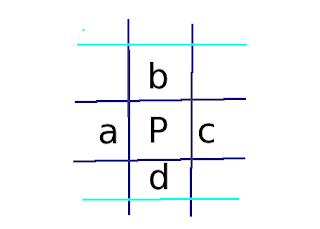# javahotel

Blog do projektu Open Source JavaHotel

## niedziela, 4 października 2009

### Math exercise and proof

Exercise from "Michał Krych, Analiza Matematyczna część 1".Assume that we have an infinite grid filled with natural numbers. Every number P and four number a,b,c,d in adjacent cells should satisfy the equation 4*P <= a + b + c + d.
Prove that all numbers in grid are equal.

Proof

Notice that grid filed with equal numbers holds statement.

Assume that there exists a grid which satisfy equation and have different numbers.

Inductive proof.

Assume that P = 1. It involves that a,b,c,d should be also equal 1 because it is the only way to satisfy equation. The same can be applied for a, b,c d and their adjacent cells and on. So having number 1 in one cell cause all grid being filled with 1. So we have to exclude number 1 from grid.

Assume that all numbers from 1 to N are excluded. Look at the cell with number N+1 and having at least one number different than N+1 in adjacent cell (assume number a). "Different" means greater then N+1 because all number less than N are excluded. But if a > N and b, c and d  >= N then a+b+c+d > N which breaks equation.

That means that all natural number are excluded from the grid and the only grid holding statement must have all number equal.